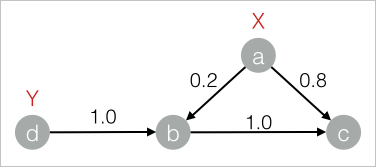Label propagation classification is a semi-supervised classification algorithm. It uses the label information of labeled nodes to predict the label information for unlabeled nodes. This topic describes the Label Propagation Classification component provided by Machine Learning Studio.

## Background information

The basic idea behind the label propagation classification algorithm is that: During algorithm execution, the labels of each node are propagated to the neighboring nodes based on the similarity between the nodes. In each step of propagation, a node updates its labels based on the labels of the neighboring nodes. A higher similarity indicates a higher labeling influence that the neighboring nodes have on the node. In this case, the labels are easy to be propagated. During label propagation, the labels of the labeled data remain unchanged. These labels serve as sources for propagation to the unlabeled data. After the iterations end, the probability distributions of similar nodes tend to be similar. These nodes can be classified into the same category. This completes the label propagation.

You can configure the component by using one of the following methods:

## Machine Learning Platform for AI console

Tab Parameter Description
Fields Settings Vertex Table: Vertex Column The vertex column in the vertex table.
Vertex Table: Label Column The vertex label column in the vertex table.
Vertex Table: Weight Column The vertex weight column in the vertex table.
Edge Table: Source Vertex Column The start vertex column in the edge table.
Edge Table: Target Vertex Column The end vertex column in the edge table.
Edge Table: Weight Column The edge weight column in the edge table.
Parameters Settings Maximum Iterations The maximum number of iterations. Default value: 30. This parameter is optional.
Damping Coefficient The damping coefficient. Default value: 0.8.
Convergence Coefficient The convergence coefficient. Default value: 0.000001.
Tuning Workers The number of vertices for parallel job execution. The parallelism level and framework communication costs increase with the value of this parameter.
Memory Size Per Worker (MB) The maximum size of memory that a single job can use. By default, the system allocates 4,096 MB for each job. If the used memory size exceeds the value of this parameter, the OutOfMemory exception is reported.

## PAI command

``````PAI -name LabelPropagationClassification
-project algo_public
-DinputEdgeTableName=LabelPropagationClassification_func_test_edge
-DfromVertexCol=flow_out_id
-DtoVertexCol=flow_in_id
-DinputVertexTableName=LabelPropagationClassification_func_test_node
-DvertexCol=node
-DvertexLabelCol=label
-DoutputTableName=LabelPropagationClassification_func_test_result
-DhasEdgeWeight=true
-DedgeWeightCol=edge_weight
-DhasVertexWeight=true
-DvertexWeightCol=label_weight
-Dalpha=0.8
-Depsilon=0.000001;``````
Parameter Required Description Default value
inputEdgeTableName Yes The name of the input edge table. No default value
inputEdgeTablePartitions No The partitions in the input edge table. Full table
fromVertexCol Yes The start vertex column in the input edge table. No default value
toVertexCol Yes The end vertex column in the input edge table. No default value
inputVertexTableName Yes The name of the input vertex table. No default value
inputVertexTablePartitions No The partitions in the input vertex table. Full table
vertexCol Yes The vertex column in the input vertex table. No default value
outputTableName Yes The name of the output table. No default value
outputTablePartitions No The partitions in the output table. No default value
lifecycle No The lifecycle of the output table. No default value
workerNum No The number of vertices for parallel job execution. The parallelism level and framework communication costs increase with the value of this parameter. Not configured
workerMem No The maximum size of memory that a single job can use. By default, the system allocates 4,096 MB for each job. If the used memory size exceeds the value of this parameter, the OutOfMemory exception is reported. 4096
splitSize No The data split size. 64
hasEdgeWeight No Specifies whether the edges in the input edge table have weights. false
edgeWeightCol No The edge weight column in the input edge table. No default value
hasVertexWeight No Specifies whether the vertices in the input vertex table have weights. false
vertexWeightCol No The vertex weight column in the input vertex table. No default value
alpha No The damping coefficient. 0.8
epsilon No The convergence coefficient. 0.000001
maxIter No The maximum number of iterations. 30

## Examples

1. Generate training data.
``````drop table if exists LabelPropagationClassification_func_test_edge;
create table LabelPropagationClassification_func_test_edge as
select * from
(
select 'a' as flow_out_id, 'b' as flow_in_id, 0.2 as edge_weight from dual
union all
select 'a' as flow_out_id, 'c' as flow_in_id, 0.8 as edge_weight from dual
union all
select 'b' as flow_out_id, 'c' as flow_in_id, 1.0 as edge_weight from dual
union all
select 'd' as flow_out_id, 'b' as flow_in_id, 1.0 as edge_weight from dual
)tmp
;
drop table if exists LabelPropagationClassification_func_test_node;
create table LabelPropagationClassification_func_test_node as
select * from
(
select 'a' as node,'X' as label, 1.0 as label_weight from dual
union all
select 'd' as node,'Y' as label, 1.0 as label_weight from dual
)tmp;``````
The following figure shows the structure of the label propagation classification graph.2. View training results.
``````+------+-----+------------+
| node | tag | weight     |
+------+-----+------------+
| a    | X   | 1.0        |
| b    | X   | 0.16667    |
| b    | Y   | 0.83333    |
| c    | X   | 0.53704    |
| c    | Y   | 0.46296    |
| d    | Y   | 1.0        |
+------+-----+------------+``````